# Math > Year 2

## Measuring & Comparing Mass in kg & g

Mass:
It is defined as a measurement of how heavy or how light something is.

How to measure the mass of an object?

We can use a weighing machine to measure the mass of an object.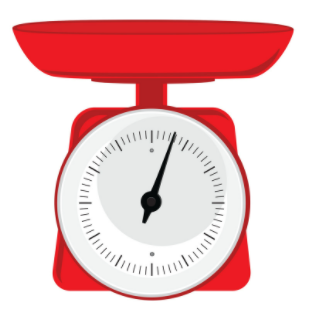Units of mass: Mass can be measured in kilograms and grams.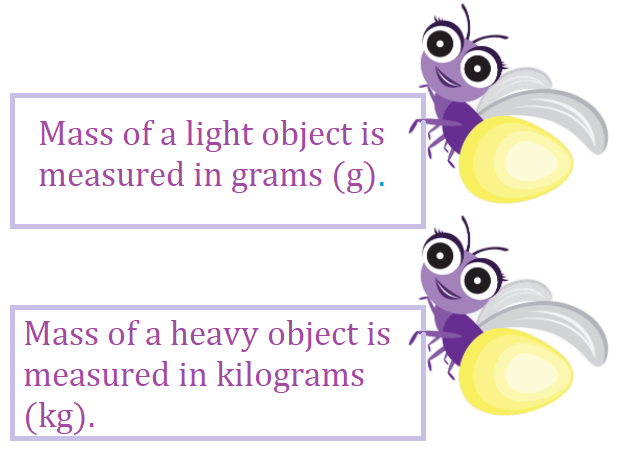1kg = 1,000g

How to record the weight of the objects?

Weight in kilograms: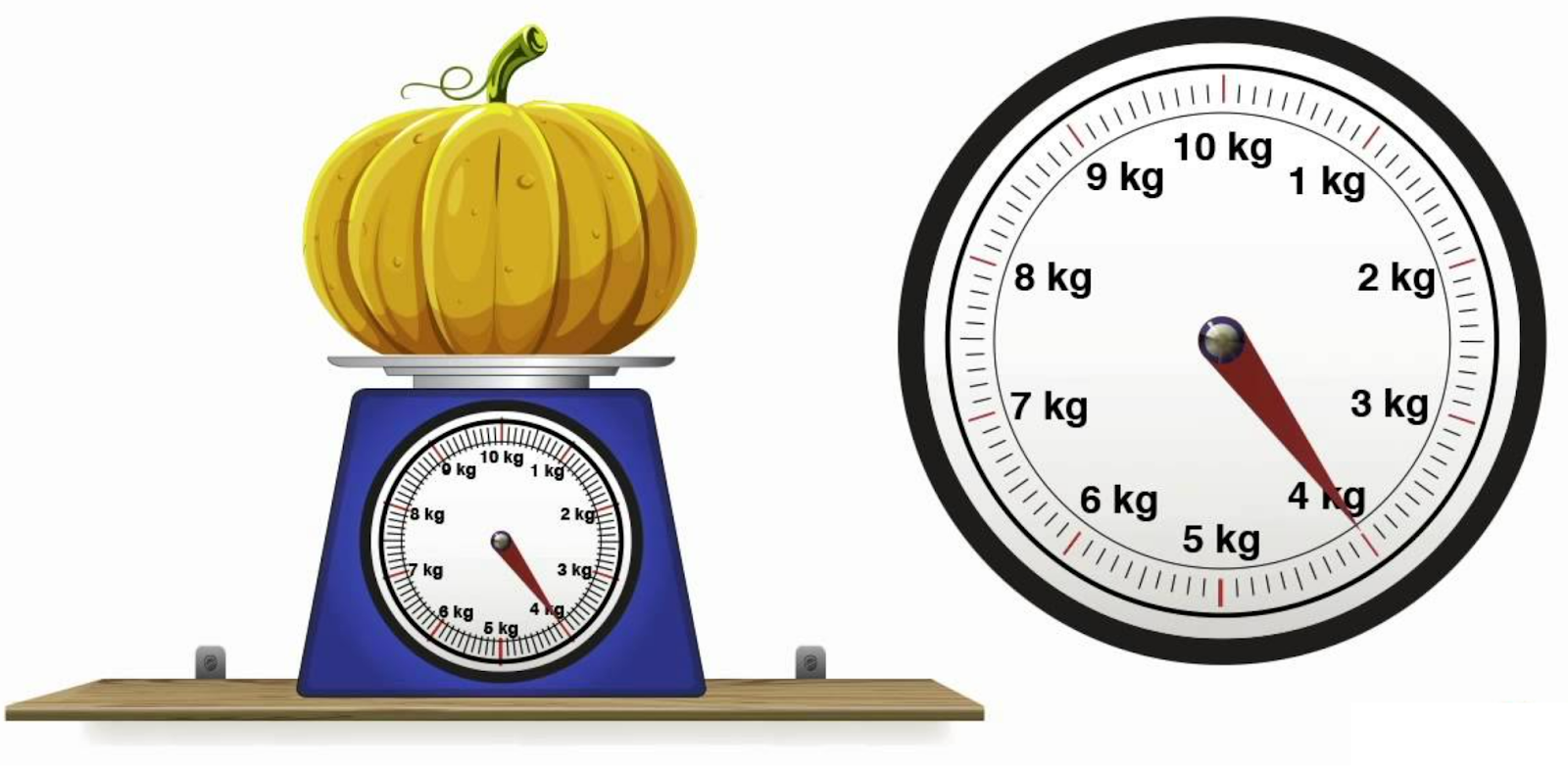We can see that the red needle is coinciding with 4 kg.

That means the weight of the pumpkin is 4 kg.

Weight in grams: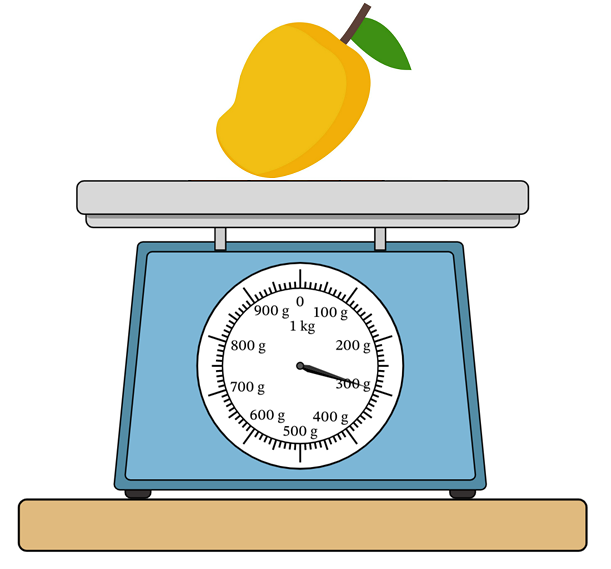We can see that the needle is pointing towards 300 g.

That means the weight of a mangos 300g.

How can we compare the masses of different objects?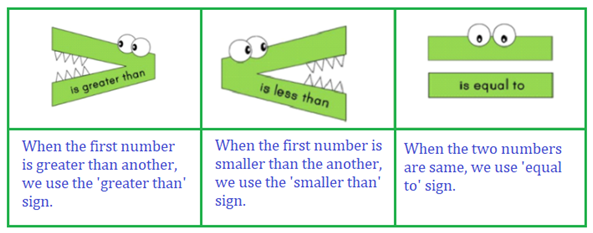Example:

Choose the correct symbol.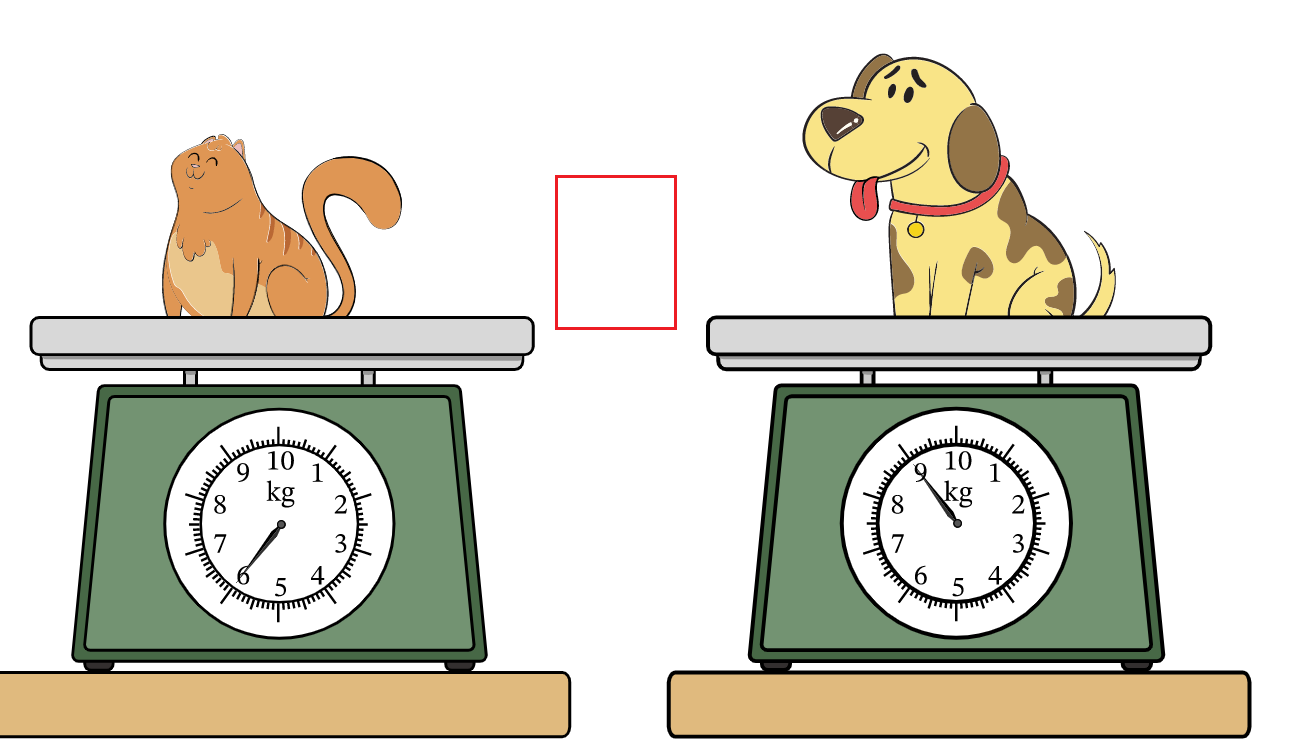Weight of cat = 6kg

Weight of dog = 9 kg

Weight of cat < Weight of dog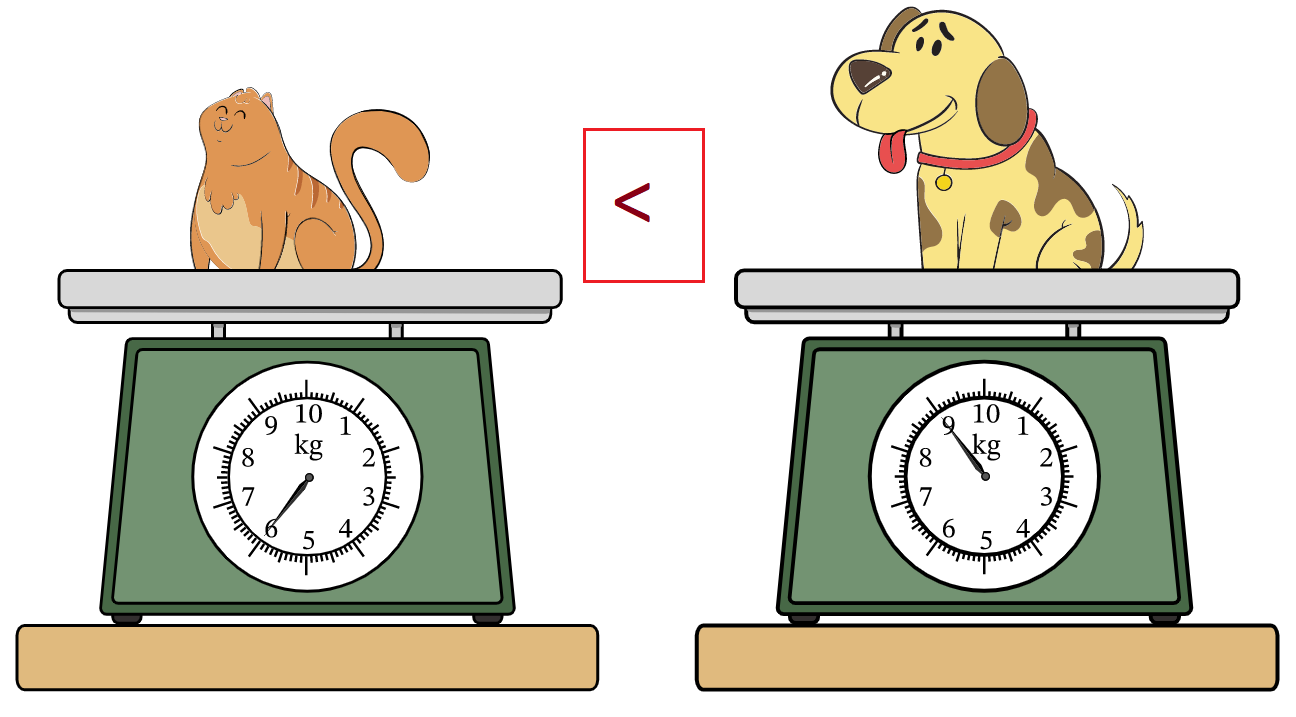Let’s try a question:

Question: Put the correct symbol.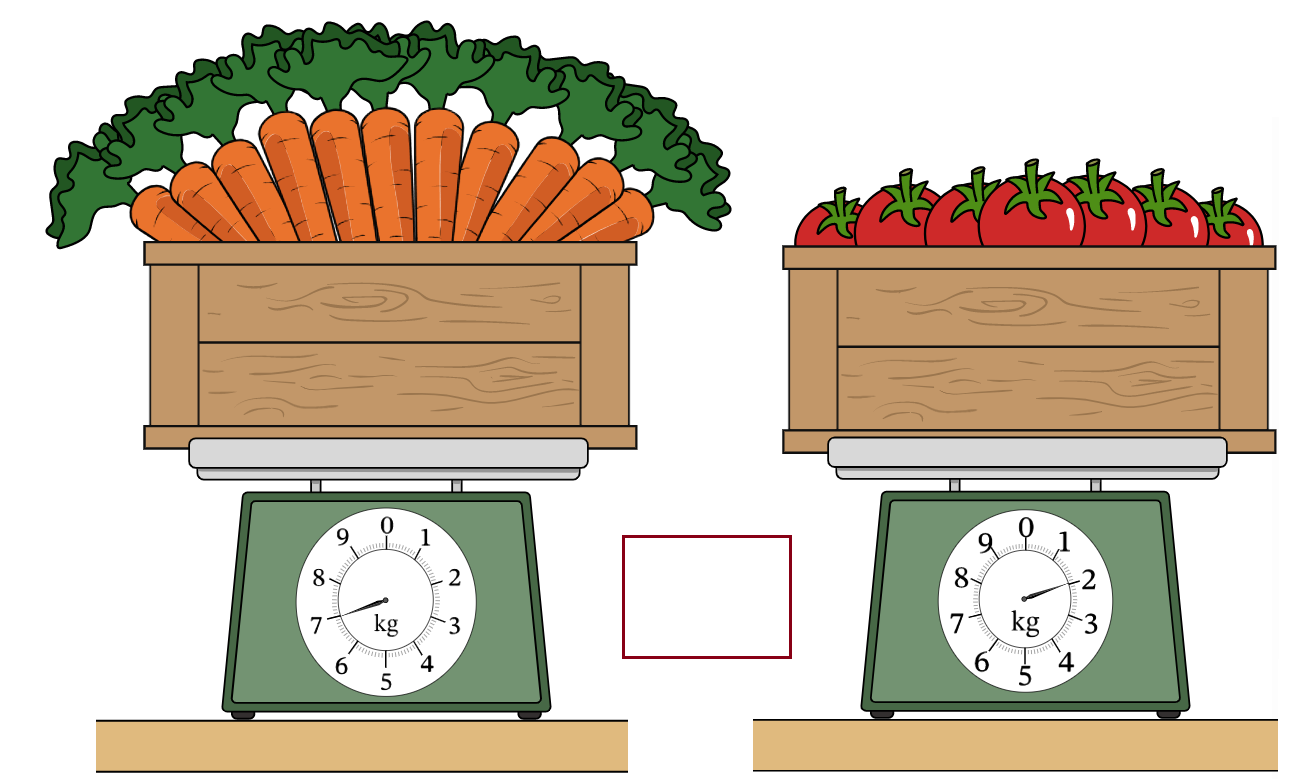Weight of the carrots = 7 kg

Weight of the tomatoes = 2 kg

7 kg > 2kg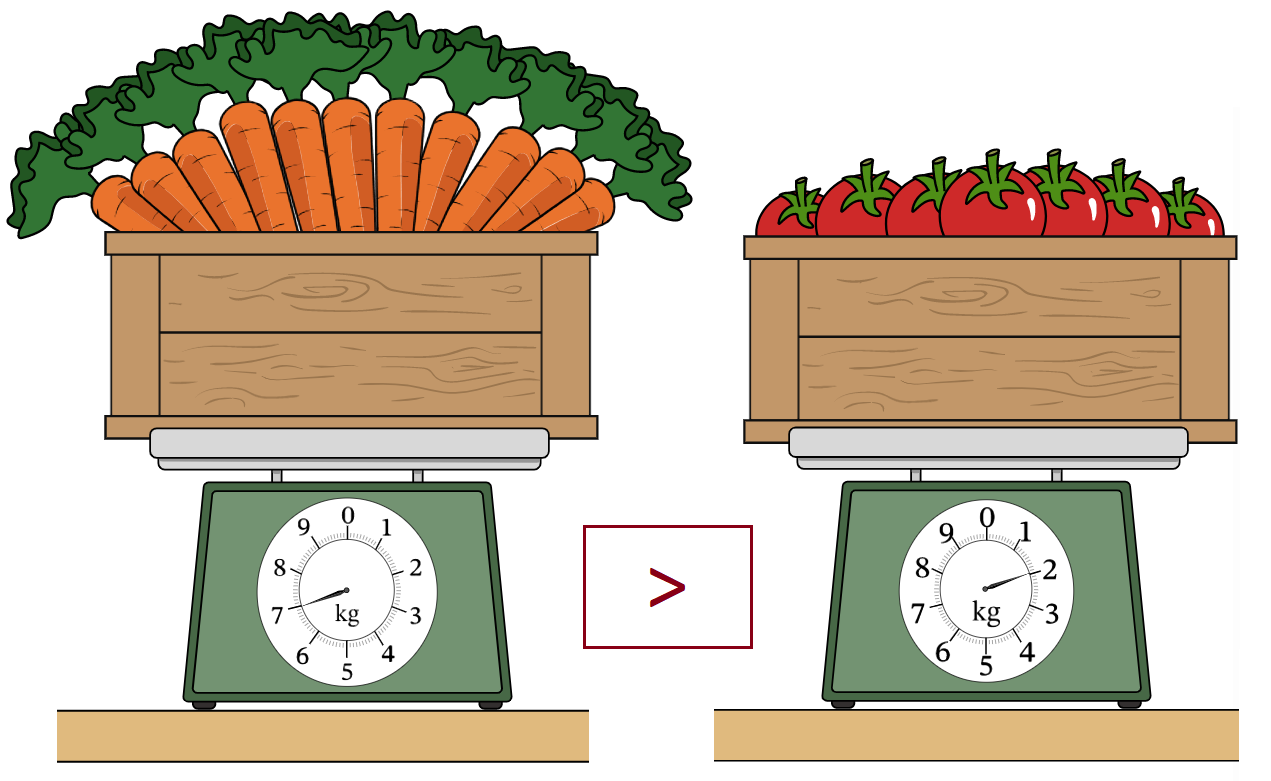//